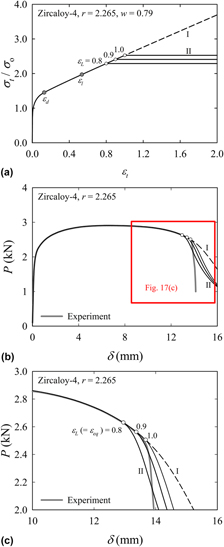Hostname: page-component-594f858ff7-hf9kg Total loading time: 0 Render date: 2023-06-08T05:35:00.068Z Has data issue: false Feature Flags: { "corePageComponentGetUserInfoFromSharedSession": true, "coreDisableEcommerce": false, "corePageComponentUseShareaholicInsteadOfAddThis": true, "coreDisableSocialShare": false, "useRatesEcommerce": true } hasContentIssue false

# On acquiring true stress–strain curves for sheet specimens using tensile test and FE analysis based on a local necking criterion

Published online by Cambridge University Press:  04 March 2014

*
a)Address all correspondence to this author. e-mail: minsist@sogang.ac.kr

## AbstractIn this study, we obtain true stress–strain (SS) curves for a sheet specimen under consideration of local necking and material anisotropy. We first extract the SS curve up to the diffuse necking point from the tensile test load–displacement data. The curve's part after the onset of diffuse necking is extrapolated by the weighted-average method proposed by Ling [Y. Ling, AMP J. Technol. 5, 37–48 (1996)]. Initiation of local necking is predicted by means of the minor-to-major strain ratio in the specimen's center. We propose a criterion to determine the strain ratio at the onset of local necking and the major strain corresponding to the strain ratio at local necking. We complete the true SS curve by cutting off the SS curve at the major strains corresponding to the local necking or apparent fracture point. Finally, the effects of material anisotropy on SS curves are discussed.

Type
Articles
Information
Journal of Materials Research , 14 March 2014 , pp. 695 - 707

## Access options

Get access to the full version of this content by using one of the access options below. (Log in options will check for institutional or personal access. Content may require purchase if you do not have access.)

## References

#### REFERENCES

Bressan, J.D. and Unfer, R.K.: Construction and validation tests of a torsion test machine. J. Mater. Process. Technol. 179, 2329 (2006).CrossRefGoogle Scholar
Cabezas, E.E. and Celentano, D.J.: Experimental and numerical analysis of the tensile test using sheet specimens. Finite Elem. Anal. Des. 40, 555575 (2004).CrossRefGoogle Scholar
Dumoulin, S., Tabourot, L., Chappuis, C., Vacher, P., and Arrieux, R.: Determination of the equivalent stress-equivalent strain relationship of a copper sample under tensile loading. J. Mater. Process. Technol. 133, 7983 (2003).CrossRefGoogle Scholar
Gelin, J.C. and Ghouati, O.: The inverse approach for the determination of constitutive equations in metal forming. Ann. CIRP 44, 189192 (1995).CrossRefGoogle Scholar
Huber, N. and Tsakmakis, C.: Determination of constitutive properties from spherical indentation data using neural networks. Part II: Plasticity with nonlinear isotropic and kinematic hardening. J. Mech. Phys. Solids 47, 15891607 (1999).CrossRefGoogle Scholar
Lee, H., Lee, J.H., and Pharr, G.M.: A numerical approach to spherical indentation techniques for material property evaluation. J. Mech. Phys. Solids 53, 20372069 (2005).CrossRefGoogle Scholar
Mirone, G.: A new model for the elastoplastic characterization and the stress-strain deformation on the necking section of a tensile specimen. Int. J. Solids Struct. 41, 35453564 (2004).CrossRefGoogle Scholar
Hyun, H.C., Lee, J.H., and Lee, H.: Mathematical expressions for stress-strain curve of metallic material. Trans. KSME 32, 2128 (2008).Google Scholar
Bridgman, P.W.: Studies in Large Plastic Flow and Fracture (McGraw Hill Book Company Inc., New York, 1952).Google Scholar
Joun, M.S., Choi, I.S., Eom, J.G., and Lee, M.C.: Finite element analysis of tensile testing with emphasis on necking. Comput. Mater. Sci. 41, 6369 (2007).CrossRefGoogle Scholar
Joun, M.S., Eom, J.G., and Lee, M.C.: A new method for acquiring true stress-strain curves over a large range of strains using a tensile test and finite element method. Mech. Mater. 40, 586593 (2008).CrossRefGoogle Scholar
Ling, Y.: Uniaxial true stress-strain after necking. AMP J. Technol. 5, 3748 (1996).Google Scholar
Tao, H., Zhang, N., and Tong, W.: An iterative procedure for determining effective stress-strain curves of sheet metals. Int. J. Mech. Mater. Des. 5, 1327 (2009).CrossRefGoogle Scholar
Zhang, K.S. and Li, Z.H.: Numerical analysis of the stress-strain curve and fracture initiation for ductile material. Eng. Fract. Mech. 49, 235241 (1994).CrossRefGoogle Scholar
Tardif, N. and Kyriakides, S.: Determination of anisotropy and material hardening for aluminum sheet metal. Int. J. Solids Struct. 49, 34963506 (2012).CrossRefGoogle Scholar
Zhang, Z.L., Hauge, M., Odegard, J., and Thaulow, C.: Determining material true stress-strain curve from tensile specimens with rectangular cross-section. Int. J. Solids Struct. 36, 34973516 (1999).CrossRefGoogle Scholar
Scheider, I., Brocks, W., and Cornec, A.: Procedure for the determination of true stress-strain curves from tensile tests with rectangular cross-section specimens. ASME J. Eng. Mater. Technol. 126, 7076 (2004).CrossRefGoogle Scholar
Hyun, H.C., Kim, M., Lee, H., and Kim, N.: Sectional FE forming limit models of Zircaloy-4 and Zirlo sheets for stamping process of spacer grids of nuclear fuel rods (2014, in preparation).Google Scholar
Hyun, H.C., Kim, M., Lee, H., and Kim, N.: Dependency of forming limit stress diagram on yield criterion in Zircaloy-4 and Zirlo Sheets (2014, in preparation).Google Scholar
ABAQUS User’s Manual, 2010. Version 6.10 (Dassault Systemes Simulia Corp., Providence, RI).
Gurson, A.L.: Continuum theory of ductile rupture by void nucleation and growth: Part-1: Yield criteria and flow rules for porous ductile media. J. Eng. Mater. Technol. 99, 215 (1977).CrossRefGoogle Scholar
Tvergaard, V. and Needleman, A.: Analysis of the cup-cone fracture in a round tensile bar. Acta Metall. 32, 157169 (1984).CrossRefGoogle Scholar
Zhang, Z.L., Ødegard, J., Sovik, O.P., and Thaulow, C.: A study on determining true stress–strain curve for anisotropic materials with rectangular tensile bars. Int. J. Solids Struct. 38, 44894505 (2001).CrossRefGoogle Scholar
Hill, R.: On discontinuous plastic state, with special reference to localized necking in thin sheets. J. Mech. Phys. Solids 1, 1930 (1952).CrossRefGoogle Scholar